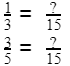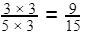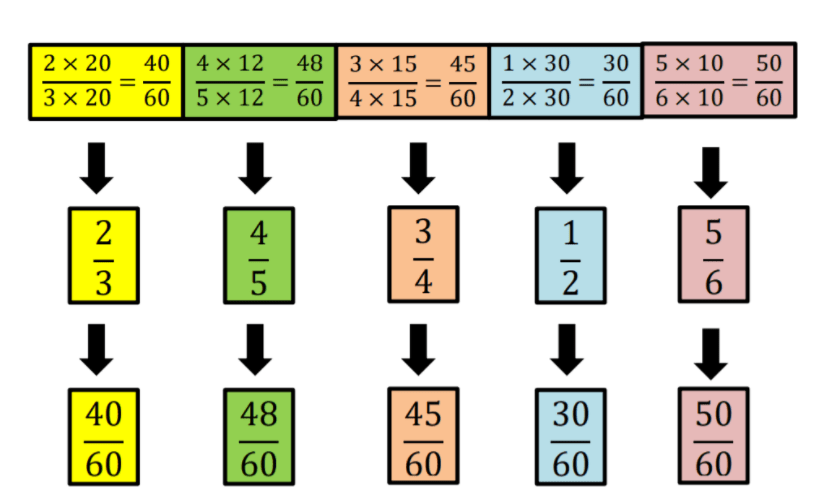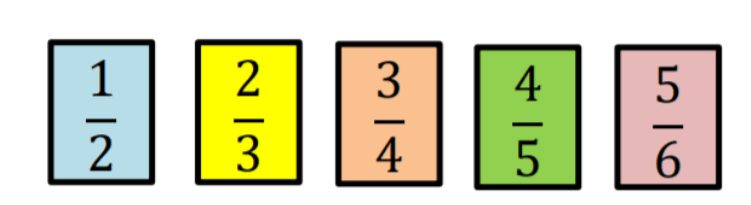# Common Denominators

 Listen to this Lesson:

In units on fractions, fifth graders learn how to list down multiples to find common denominators, how to determine common denominators shared by fractions using prime factorization, as well as express fractions with their equivalent fractions sharing a common denominator.

A good understanding of common denominators is essential for students to be able to add and subtract fractions with unlike denominators. And yet, many students end up with gaps in their knowledge of common denominators.

To avoid this and to help out, we’ve compiled a list of teaching ideas and fun activities on this topic that will have your students master common denominators in no time! Read on and learn more.## How to Teach Common Denominators

### What Is a Denominator?

Start your lesson on common denominators by first reminding students what a denominator represents. We know that fractions are made up of a numerator and a denominator. So the numerator is the top number and the denominator is the bottom number in a given fraction.

For example, in 4⁄5 the numerator is 4 and the denominator is 5; in 1⁄8 the numerator is 1 and the denominator is 8; in 20, the numerator is 2 and the denominator is 1. Add that the horizontal line that separates the numerator and the denominator is called a ‘fraction bar’.

### What Are Common Denominators?

Explain that when we have two or more fractions, we can express them with the same denominators. When fractions have the same denominators, we can say that these fractions have common denominators.

Provide a few examples. For instance, 1⁄5 and 3⁄5 have a common denominator, which is 5; 4⁄15 and 2⁄15 have a common denominator 15; 7⁄2 and 3⁄2 have a common denominator 2, etc.

### Finding Common Denominators

Sometimes, fractions don’t have a common denominator and we wish to find one. In cases like these, point out that we need to master the skill of finding the least common multiple of numbers using the following methods:

• listing method
• prime factorization

#### Finding the Least Common Multiple by Listing Method

Explain to students how to use the listing method first. Point out that when we have two numbers (or denominators), we can simply list down each of their multiples and determine the common multiples they share.

Remind students that a multiple is a number that we can divide by another number without any remainder. For instance, 20 is a multiple of 4 and 5, because 20 divided by 4 is 5 (no remainder) and 20 divided by 5 is 4 (again no remainder).

Provide an example of the listing method. Let’s say we want to express 3⁄5 and 1⁄3 as fractions with common denominators. Point out that we’ll start by listing the multiples of the denominators, that is, 3 and 5:

Multiples of 3: 3, 6, 9, 12, 15, 18, 21, 24…

Multiples of 5: 5, 10, 15, 20, 25, 30, 35, 40…

We’ll observe the list of multiples and try to find the least common multiple or LCM, which is the smallest number that is a multiple of two or more numbers, also known as the lowest common denominator (LCD).

We can easily observe that this is 15. So 15 represents the least common multiple (LCM) shared by 3 and 5. Add that we can perform the same process when finding common multiples shared by three numbers or more.

#### Finding Least Common Multiple by Prime Factorization

Point out that sometimes, listing down multiples to find the least common multiple can be time-consuming, especially for numbers with no small common multiple. Another way of finding the smallest common multiple mentioned above is prime factorization.

Remind students that prime numbers are numbers that are divisible only by themselves (2, 3, 5, 7, 11, 13, 17, etc.). Then, define prime factors as factors that are prime numbers, and prime factorization as finding which prime numbers multiply together to get the original number.

Provide an example of this process. For instance, let’s say we want to find the prime factors of 6 and 20. We can express 6 and 20 as products of their prime factors. We’ll also try to match the prime numbers vertically and bring down the prime numbers in each column.

Then, we’ll simply multiply the prime numbers and the product will represent the LCM:So the least common multiple shared by 6 and 20 is 60.

Point out that we can also apply prime factorization to the above example, where we were trying to find the common denominators of 3⁄5 and 1⁄3. By expressing 3 and 5 as products of their prime factors, we’ll get the following:

3 = 3

5 = 5

This means that these two numbers are expressed as products of one prime factor each, so the only thing left to do is multiply these prime factors, i.e. 3 and 5. This results in 15, which represents the least common multiple shared by 3 and 5, or their common denominator.### Writing Fractions With Common Denominators

After determining the common denominators shared by two or more fractions, we can then express them as fractions with the same denominators. Here, we are using the concept of ‘equivalent fractions’.

Remind students that equivalent fractions are fractions that are equal and represent the same parts, but have different values for their numerators and denominators. For example, 1⁄2, 2⁄4 and 4⁄8 represent the same amount or parts, so they are equivalent fractions.

For instance, let’s say that we wish to represent 3⁄5 and 1⁄3 as fractions with common denominators. Since we already saw that these two fractions share a common denominator of 15, we can express them as fractions with 15 as the denominator. In other words:In order to find the missing numerator in 3⁄5= ?⁄15, point out that first, we’ll determine the factor we need to change 5 to 15. As 15 divided by 5 is 3, this means we’ll need to multiply 5 by 3 so that we end up with 15 in the denominator.

However, highlight that in order for the value of the fraction to remain unchanged (and thus obtain an equivalent fraction), we’ll have to multiply the numerator, that is, 3, by the same number by which we multiply the denominator, i.e. 3 again. This will produce the following:Add that we’ll perform the same process with the other fraction to find the missing numerator in 1⁄3= ?⁄15. We have to determine the factor we need to change 3 to 15. As 15 divided by 3 is 5, we need to multiply 3 by 5 to get 15 as a denominator.

To produce an equivalent fraction nevertheless, point out that again we’ll have to multiply the numerator as well by the same number by which we multiply the denominator. That is, we have to multiply 1 by 5 also:### Arranging Fractions

#### Arranging Similar Fractions

Arranging fractions with the same denominators in ascending or descending order is very easy. If fractions are similar, the bigger the numerator, the fraction becomes greater. And of course, the smaller the numerator, the fraction becomes lesser.

#### Arranging Dissimilar Fractions

Explain to students that if the given fractions have different denominators and are dissimilar, we have to use a special method to arrange them. This is where finding common denominators and expressing them as fractions with the same denominators gets in the picture.

Provide an example. Let’s say we wish to arrange the following factors in ascending order:Explain that we can use the listing method or prime factorization to achieve this. The important thing is that we’re trying to express all the above factors as fractions with common denominators.

By using the prime factorization method, we’ll start by expressing all denominators, i.e. 3, 5, 4, 2, and 6 as products of their prime factors. We’ll also try to match the prime numbers vertically and bring down the prime numbers in each column.

3 = 3

5 = 5

4 = 2 x 2

2 = 2

6 = 3 x 2

LCM = 3 x 5 x 2 x 2 = 60. So the common denominator is 60.After determining the common denominator, point out that we’ll express the above fractions as fractions with the same denominators:Finally, now that all the fractions have the same denominator and are transformed into similar fractions, we can easily arrange them in ascending order by following the rule of ‘the bigger the numerator, the fraction becomes greater’. Finally, this will result in:If you have the technical possibilities in your classroom, you can also enrich your lesson on common denominators with multimedia materials, such as videos. Videos can also be beneficial if you’re a homeschooling parent.

For instance, use this video to introduce the concept of common denominators. The video also contains step-by-step instructions on how to 2⁄8 and 5⁄6 into fractions that have the same denominator.

The video presents both methods of finding common denominators, that is, finding the least common multiple of numbers by listing as well as finding the least common multiple of numbers by using prime factorization.

Finally, this video contains simple guidelines on how to arrange fractions with the same denominator, whereas this video is an excellent resource on illustrating how to arrange dissimilar fractions from least to greatest (in ascending order).

## Activities to Practice Common Denominators

### Snowball FightThis is a fun game that allows students to practice finding the least common multiple (LCM), and thus apply their knowledge of common denominators. To implement this game in your classroom, make sure that you have enough technical devices for all students.

Divide students into pairs and provide instructions. Students in each pair play individually. They are presented with different numbers where they have to find the LCM and participate in the snowball fight.

If they answer incorrectly too many times, they might get too cold to play. In the end, each player compares their results with the other student in their pair and discusses which exercises were more difficult and why.

### Common Denominator War

This game will help students practice finding the least common multiple (LCM) and thus finding common denominators. To implement this game in your classroom, you’ll need colorful index cards and some markers.

Start by writing numbers on one stack of index cards (for example, use some 20 cards in one stack). The numbers should mainly be random numbers from 1 to 20, but you can also include some bigger numbers with a lot of factors, such as 30 or 24. Also, don’t use prime numbers.

Pair students up and hand out one stack of cards. Divide the stack between the two students, so that each student gets around 10. The cards remain face down until you give students the signal to start playing. Provide instructions for the game.

Both students in each pair shout out ‘denominator war’ and draw the top card from their stack. They then place the card on the table, so that the other student can see it. Both students then try to find the least common denominator of the two numbers as fast as they can.

The student that manages to calculate the LCM of the two numbers first, wins that round and takes both cards. The game ends once a student doesn’t have any cards left in his pile. The winner is then the student with all cards.

### Arranging Fractions Game

In this simple game, students will get to practice arranging dissimilar fractions by using either the listing method or prime factorization. In doing so, they will practice expressing fractions as fractions with common denominators.

To implement this game in your classroom, you’ll need index cards (the more colorful the better, as this would make the game more visually stimulating), and markers. Use the markers to write different dissimilar fractions on the cards (one fraction per card).

Divide students into groups of 3, 4 and hand out a pile of cards (ex: you could use 10 cards per group). Students in each group arrange the cards in ascending order, by applying common denominators.

Provide a few minutes for students to complete ordering the cards. Then, open space for discussion and reflection. What was the common denominator in each group? After transforming them into similar fractions, which rule did students use for arranging the fractions?

### Least Common Denominator Game

In this online game, students will practice finding the least common denominator or the least common multiple, by using the listing method or prime factorization. The only materials needed for this game are suitable technical devices.

Divide students into teams of 3 and provide instructions. Students click on a question that can get them from 100 to 500 points (depending on the difficulty of the question), where they are asked to find the least common denominator for the given dissimilar fractions.

If they answer correctly, they add the number of points earned in the field that represents their team, after which another player selects a question and the process is repeated. In the end, the player that has the most points wins the game.

## Before You Leave…

If you enjoyed these ideas and activities for teaching common denominators, you’ll want to check out our lesson that’s dedicated to this topic! So if you need guidance to structure your class and teach it, sign up for our emails to receive loads of free content!

Feel free to also check out our blog – you’ll find plenty of awesome resources that you can use in your class! And if you’re ready to become a member, simply join our Math Teacher Coach community!